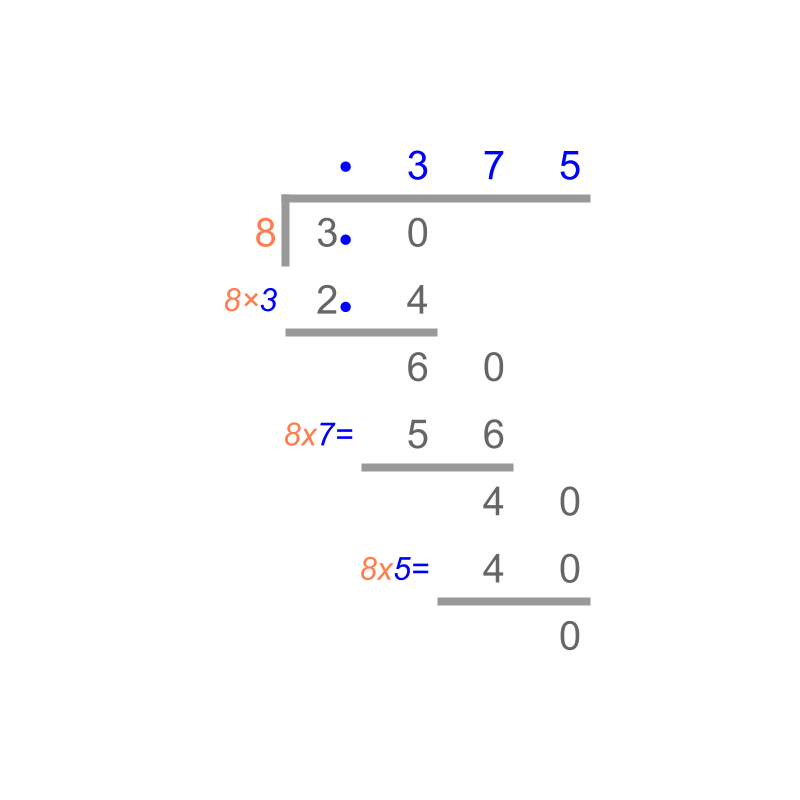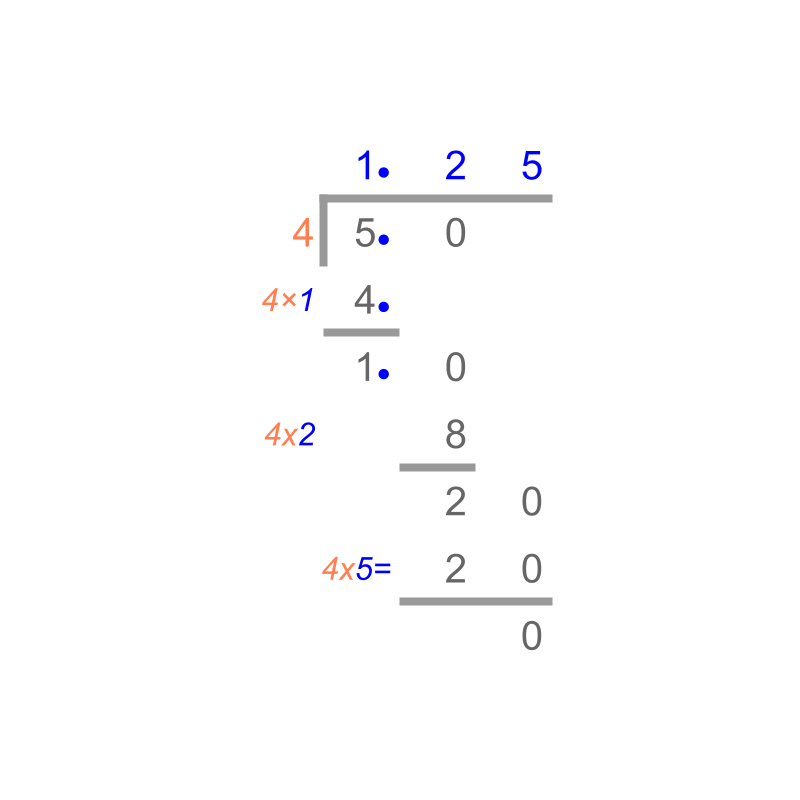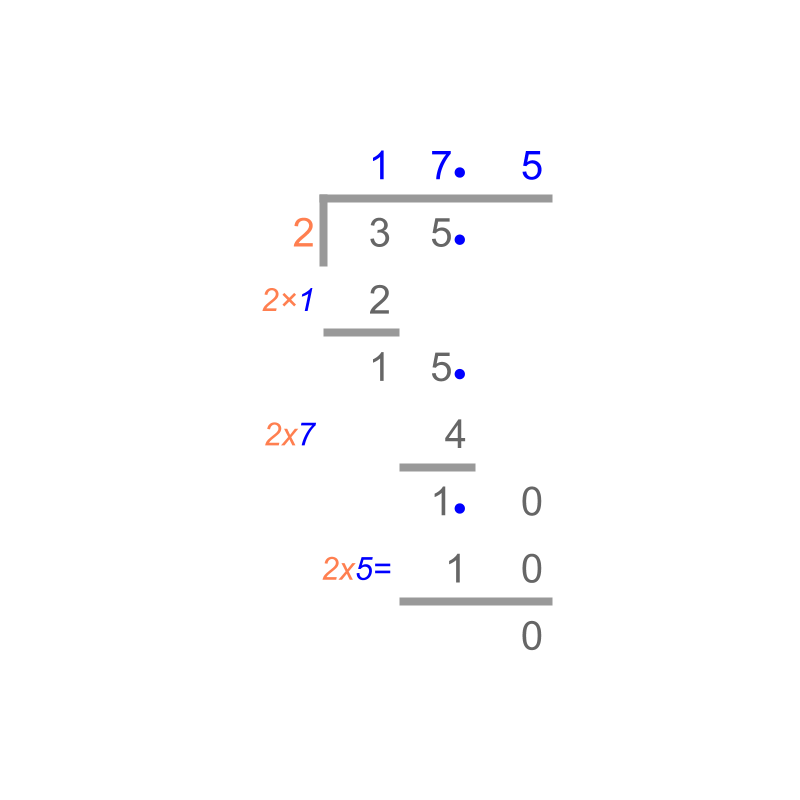maths > decimals

Conversion of Fraction and Decimals

what you'll learn...

overview

•  fractions to decimals by long division method

•  decimals to fractions by simplifying the equivalent fraction

convert

Decimal numbers are standardized fractions.

The decimal representation of $\frac{1}{2}$$\frac{1}{2}$ is "$0.5$$0.5$"
By first principles, $\frac{1}{2}$$\frac{1}{2}$ is converted to the equivalent fraction $\frac{5}{10}$$\frac{5}{10}$, which is $0.5$$0.5$ in decimals.

Note: By first principles, decimals are fractions with denominator $10$$10$, $100$$100$, $1000$$1000$ etc. Since the denominator is standardized, it is dropped and is represented with a decimal point.

The fraction $\frac{3}{8}$$\frac{3}{8}$ is "$0.375$$0.375$" in decimals.

Considering converting the fraction $\frac{3}{8}$$\frac{3}{8}$ to decimal.

By first principles, the fraction has to be converted into an equivalent fraction having denominator one of $10$$10$, $100$$100$, $1000$$1000$, etc.

$8$$8$ is not a factor of $10$$10$. So the fraction cannot be converted to an equivalent fraction with denominator $10$$10$

$8$$8$ is not a factor of $100$$100$ either. So the fraction cannot be converted to an equivalent fraction with denominator $100$$100$.

$8$$8$ is a factor of $1000=8×125$$1000 = 8 \times 125$. So, the denominator and numerator are multiplied by $125$$125$.

$\frac{3}{8}=\frac{3×125}{8×125}=\frac{375}{1000}$$\frac{3}{8} = \frac{3 \times 125}{8 \times 125} = \frac{375}{1000}$

$\frac{375}{1000}=\frac{3}{10}+\frac{7}{100}+\frac{5}{1000}$$\frac{375}{1000} = \frac{3}{10} + \frac{7}{100} + \frac{5}{1000}$

$=0.375$$= 0.375$ in decimal.A simplified procedure is devised: By long division method, the decimal is calculated. This is shown in the figure.

Converting a Fraction to a Decimal -- simplified procedure : A fraction is converted to the equivalent decimal by long division method.

examples

Convert $\frac{5}{4}$$\frac{5}{4}$ into decimal.
The answer is "$1.25$$1.25$".

By long division method,Convert $\frac{35}{2}$$\frac{35}{2}$ into decimal.
The answer is "$17.5$$17.5$".

By long division method,We learned that the fractions can be converted to decimals. Similarly, decimals can be converted to fractions too.

Convert $6.75$$6.75$ in to the equivalent fraction
The answer is "$6\frac{3}{4}$$6 \frac{3}{4}$"
$6.75$$6.75$
$=6\frac{75}{100}$$= 6 \frac{75}{100}$
$=6\frac{3}{4}$$= 6 \frac{3}{4}$

summary

Converting a Fraction to a Decimal -- simplified procedure : A fraction is converted to the equivalent decimal by long division method.Converting a Decimal to a Fraction : The decimal is represented in its equivalent fraction form and the fraction is simplified.
eg: $6.75$$6.75$
$=6\frac{75}{100}$$= 6 \frac{75}{100}$
$=6\frac{3}{4}$$= 6 \frac{3}{4}$

Outline